Courses

# NCERT Solutions Chapter 10 (Part - 1) - Mensuration, Maths, Class 6 | EduRev Notes

## Class 6 : NCERT Solutions Chapter 10 (Part - 1) - Mensuration, Maths, Class 6 | EduRev Notes

The document NCERT Solutions Chapter 10 (Part - 1) - Mensuration, Maths, Class 6 | EduRev Notes is a part of the Class 6 Course Mathematics (Maths) Class 6.
All you need of Class 6 at this link: Class 6

Exercise 10.1

Question 1

Find the perimeter of each of the following figures: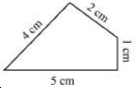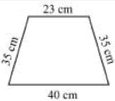(a) (b)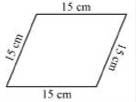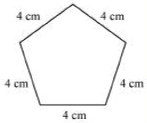(c) (d)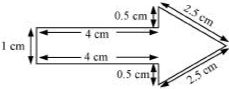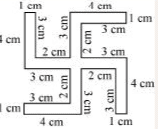(a) Perimeter = Sum of all the sides = 4 cm + 2 cm + 1 cm + 5 cm = 12 cm

(b) Perimeter = Sum of all the sides = 23 cm + 35 cm + 40 cm + 35 cm = 133 cm

(c) Perimeter = Sum of all the sides = 15 cm + 15 cm + 15 cm + 15 cm = 60 cm

(d) Perimeter = Sum of all the sides = 4 cm + 4 cm + 4 cm + 4 cm + 4 cm = 20 cm

(e) Perimeter = Sum of all the sides 1 cm + 4 cm + 0.5 cm + 2.5 cm + 2.5 cm + 0.5 cm + 4 cm = 15 cm

(f) Perimeter = Sum of all the sides = 4 cm + 1 cm + 3 cm + 2 cm + 3 cm + 4 cm + 1 cm + 3 cm + 2 cm + 3 cm + 4 cm + 1 cm + 3 cm + 2 cm + 3 cm + 4 cm + 1 cm + 3 cm + 2 cm + 3 cm = 52 cm

Question 2

The lid of a rectangular box of sides 40 cm by 10 cm is sealed all round with tape. What is the length of the tape required?

Answer 2: Total length of tape required = Perimeter of rectangle

= 2 (40 + 10)

= 2 x 50

= 100 cm

= 1 m

Thus, the total length of tape required is 100 cm or 1 m.

Question 3

A table-top measures 2 m 25 cm by 1 m 50 cm. What is the perimeter of the table-top?

Length of table top = 2 m 25 cm = 2.25 m

Breadth of table top = 1 m 50 cm = 1.50 m

Perimeter of table top = 2 x (length + breadth)

= 2 x (2.25 + 1.50)

= 2 x 3.75

= 7.50 m

Thus, the perimeter of table top is 7.5 m.

Question 4

What is the length of the wooden strip required to frame a photograph of length and breadth 32 cm and 21 cm respectively?

Answer 4: Length of wooden strip = Perimeter of photograph

Perimeter of photograph = 2 x (length + breadth)

= 2 (32 + 21)

= 2 x 53 cm

= 106 cm

Thus, the length of the wooden strip required is equal to 106 cm.

Question 5

A rectangular piece of land measures 0.7 km by 0.5 km. Each side is to be fenced with 4 rows of wires. What is the length of the wire needed?

Since the 4 rows of wires are needed.

Therefore the total length of wires is equal to 4 times the perimeter of rectangle.

Perimeter of field = 2 x (length + breadth)

= 2 x (0.7 + 0.5)

= 2 x 1.2

= 2.4 km

= 2.4 x 1000 m

= 2400 m

Thus, the length of wire = 4 x 2400 = 9600 m = 9.6 km

Question 6

Find the perimeter of each of the following shapes:

(a) A triangle of sides 3 cm, 4 cm and 5 cm.

(b) An equilateral triangle of side 9 cm.

(c) An isosceles triangle with equal sides 8 cm each and third side 6 cm.

(a) Perimeter of ΔABC

= AB + BC + CA

= 3 cm + 5 cm + 4 cm

= 12 cm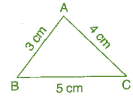(b) Perimeter of equilateral ABC

= 3 x side

= 3 x 9 cm

= 27 cm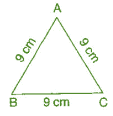(c) Perimeter of ΔABC

= AB + BC + CA

= 8 cm + 6 cm + 8 cm

= 22 cm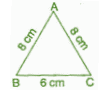Question 7

Find the perimeter of a triangle with sides measuring 10 cm, 14 cm and 15 cm.

Perimeter of triangle = Sum of all three sides

= 10 cm + 14 cm + 15 cm = 39 cm

Thus, the perimeter of triangle is 39 cm

Question 8

Find the perimeter of a regular hexagon with each side measuring 8 m.

Perimeter of Hexagon = 6 x length of one side

= 6 x 8 m = 48 m

Thus, the perimeter of hexagon is 48 m.

Question 9

Find the side of the square whose perimeter is 20 m.

Perimeter of square = 4 x side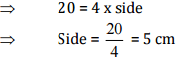Thus, the side of square is 5 cm.

Question 10

The perimeter of a regular pentagon is 100 cm. How long is its each side?

Perimeter of regular pentagon = 100 cm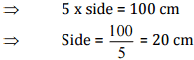Thus, the side of regular pentagon is 20 cm.

Question 11

A piece of string is 30 cm long. What will be the length of each side if the string is used to form:

(a) a square?

(b) an equilateral triangle?

(c) a regular hexagon?

Answer 11: Length of string = Perimeter of each figure

(a) Perimeter of square = 30 cm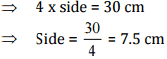Thus, the length of each side of square is 7.5 cm.

(b) Perimeter of equilateral triangle = 30 cm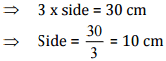Thus, the length of each side of equilateral triangle is 10 cm.

(c) Perimeter of hexagon = 30 cm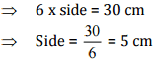Thus, the side of each side of hexagon is 5 cm.

Question 12

Two sides of a triangle are 12 cm and 14 cm. The perimeter of the triangle is 36 cm. What is its third side?

Let the length of third side be x cm.

Length of other two side are 12 cm and 14 cm.

Now, Perimeter of triangle = 36 cm

⇒ 12 +14 + x = 36
⇒ 26 + x= 36
⇒ x = 36- 26
⇒ x = 10 cm

Thus, the length of third side is 10 cm

Question 13

Find the cost of fencing a square park of side 250 m at the rate of Rs 20 per metre.

Side of square = 250 m

Perimeter of square = 4 x side

= 4 x 250

= 1000 m

Since, cost of fencing of per meter = Rs 20

Therefore, the cost of fencing of 1000 meters = 20 x 1000 = Rs20,000

Question 14

Find the cost of fencing a rectangular park of length 175 m and breadth 125 m at the rate of Rs 12 per metre.

Length of rectangular park = 175 m

Breadth of rectangular park = 125 m

Perimeter of park = 2 x (length + breadth)

= 2 x (175 + 125)

= 2 x 300 = 600 m

Since, the cost of fencing park per meter = Rs 12

Therefore, the cost of fencing park of 600 m = 12 x 600 = Rs 7,200

Question 15

Sweety runs around a square park of side 75 m. Bulbul runs around a rectangular park with length 60 m and breadth 45 m. Who covers less distance?

Distance covered by Sweety = Perimeter of square park

Perimeter of square = 4 x side

= 4 x 75 = 300 m

Thus, distance covered by Sweety is 300 m.

Now, distance covered by Bulbul = Perimeter of rectangular park

Perimeter of rectangular park = 2 x (length + breadth)

= 2 x (60 + 45)

= 2 x 105 = 210 m

Thus, Bulbul covers the distance of 210 m and Bulbul covers less distance.

Question 16

What is the perimeter of each of the following figures? What do you infer from the answers?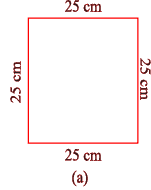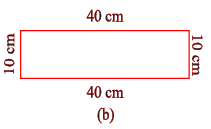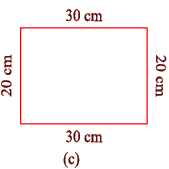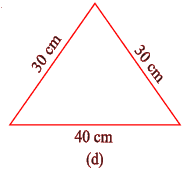(a) Perimeter of square

= 4 x side

= 4 x 25 = 100 cm

(b) Perimeter of rectangle

= 2 x (length + breadth) = 2 x (40 + 10) = 2 x 50 = 100 cm

(c) Perimeter of rectangle

= 2 x (length + breadth)

= 2 x (30 + 20) = 2 x 50 = 100 cm

(d) Perimeter of triangle = Sum of all sides

= 30 cm + 30 cm + 40 cm = 100 cm

Thus, all the figures have same perimeter.

Question 17

Avneet buys 9 square paving slabs, each with a side of 1/2 m. He lays them in the form of a square.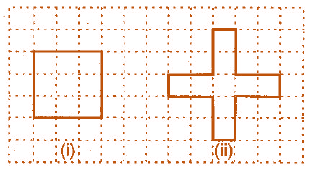(a) What is the perimeter of his arrangement [Fig (i)]?

Solution.

The arrangement so formed in Fig (i) is a square of side equal to 3 × side of the square slab

∴ Perimeter of his arrangement in Fig (i)
= 4 × ( 3 × side of the square slab)

= 4 × ( 3 × 0.5) m

= 4 × 1.5 m

= 6 m
(b) Shari does not like his arrangement. She gets him to lay them out like a cross. What is the perimeter of her arrangement [(Fig (ii)]?

Solution.

The cross arrangement so formed in Fig (ii) by 9 square slabs, has perimeter, which consists of 20 length segments each equal to side of a square slab i.e. 0.5 m

∴ Perimeter of her arrangement in Fig (ii)
= 20 × ( side of the square slab)

= 20 × 0.5 m

= 10 m
(c) Which has greater perimeter?

Solution. The Cross arrangement has greater perimeter

(d) Avneet wonders if there is a way of getting an even greater perimeter. Can you find a way of doing this? (The paving slabs must meet along complete edges i.e. they cannot be broken.)

Solution. No, there is no way of getting perimeter greater than 10 m from any other possible arrangement formed by 9 square slabs when placed edge by edge completely.

Offer running on EduRev: Apply code STAYHOME200 to get INR 200 off on our premium plan EduRev Infinity!

## Mathematics (Maths) Class 6

185 videos|229 docs|43 tests

,

,

,

,

,

,

,

,

,

,

,

,

,

,

,

,

,

,

,

,

,

,

,

,

,

,

,

;